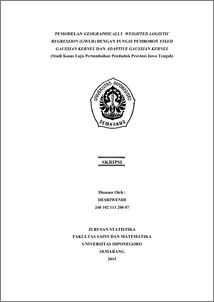# PEMODELAN GEOGRAPHICALLY WEIGHTED LOGISTIC REGRESSION (GWLR) DENGAN FUNGSI PEMBOBOT FIXED GAUSSIAN KERNEL DAN ADAPTIVE GAUSSIAN KERNEL (Studi Kasus Laju Pertumbuhan Penduduk Provinsi Jawa Tengah)

DESRIWENDI, DESRIWENDI (2015) PEMODELAN GEOGRAPHICALLY WEIGHTED LOGISTIC REGRESSION (GWLR) DENGAN FUNGSI PEMBOBOT FIXED GAUSSIAN KERNEL DAN ADAPTIVE GAUSSIAN KERNEL (Studi Kasus Laju Pertumbuhan Penduduk Provinsi Jawa Tengah). Undergraduate thesis, FSM Universitas Diponegoro.Preview
PDF
965Kb

## Abstract

The Population Growth Rate (PGR) that are not controlled will have a negative impact on the various social-economic problems such as increased poverty, crime, and so forth. Factors contributing to the population growth rate of uncontrolled allegedly various between Regency/City. Geographically Weighted Logistic Regression (GWLR) is a local form of the logistic regression where geographical factors considered. This study will analyze the factors that affect the population growth rate of Central Java Province using logistic regression and GWLR with a weighting function of Fixed Gaussian Kernel and Adaptive Gaussian Kernel. The results showed that GWLR model with a weighting function of Adaptive Gaussian Kernel better than logistic regression model and GWLR model with a weighting function of Fixed Gaussian Kernel because it has the smallest Akaike Information Criterion (AIC) value with the classification accuracy is 82.8 %. Keywords : PGR, Logistic Regression, Fixed Gaussian Kernel, Adaptive Gaussian Kernel, GWLR, AIC.

Item Type: Thesis (Undergraduate) H Social Sciences > HA Statistics Faculty of Science and Mathematics > Department of Statistics 47156 Mr Hasbi Yasin 22 Dec 2015 14:14 22 Dec 2015 14:14

Repository Staff Only: item control page